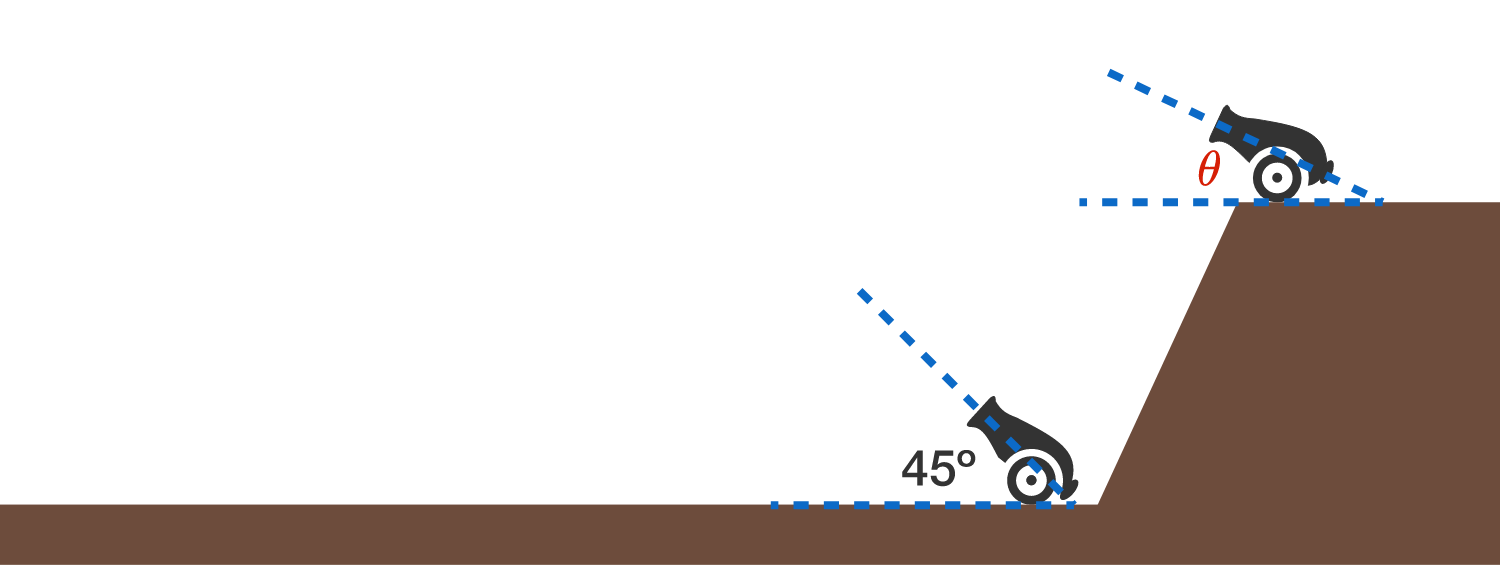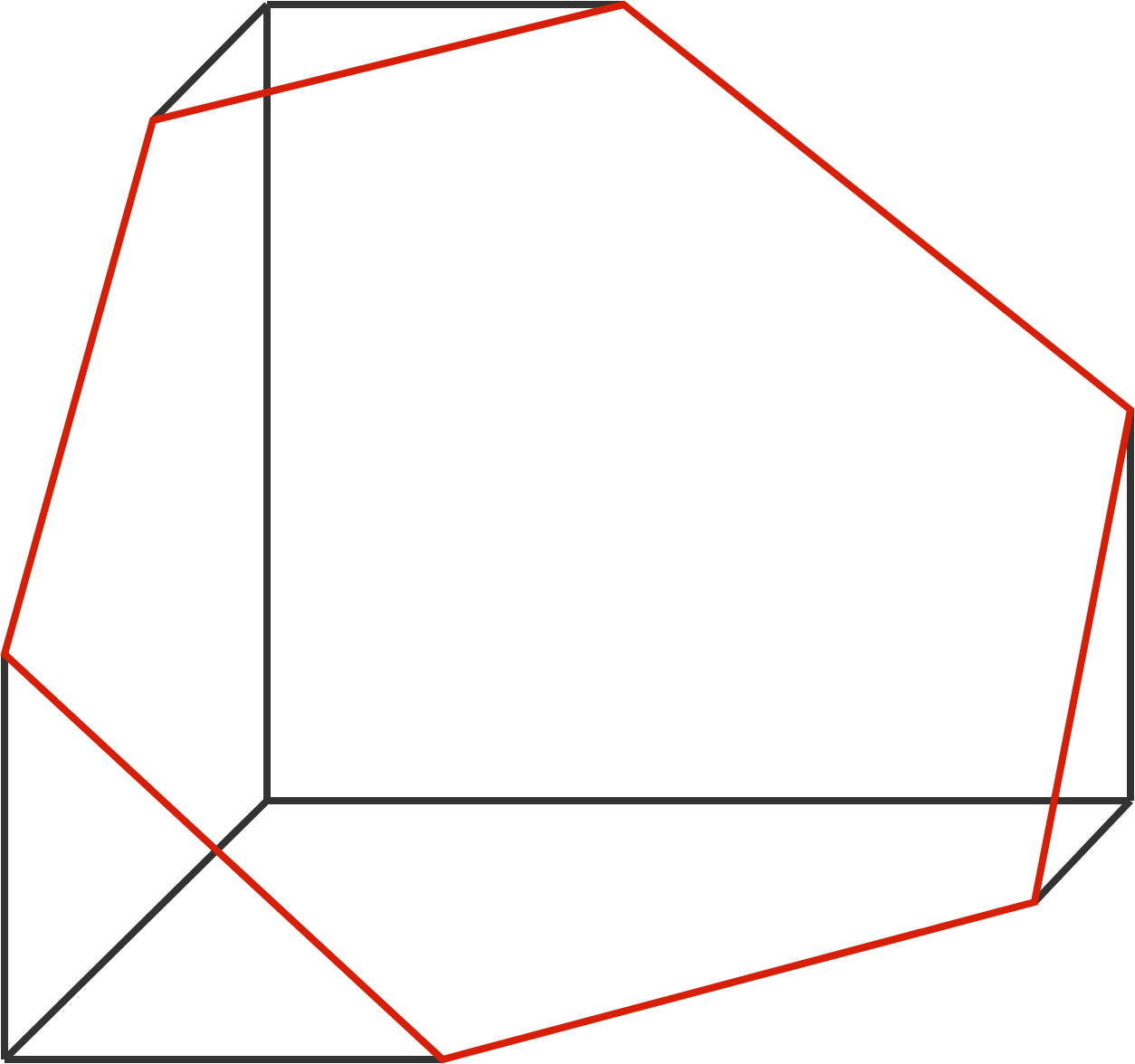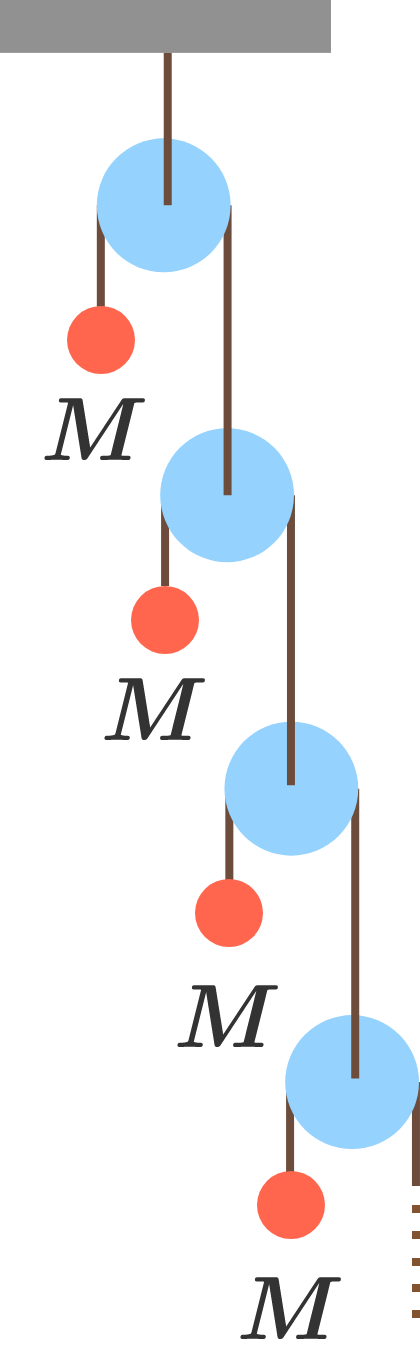# Problems of the Week

Contribute a problem

# 2018-07-30 Intermediate

When a cannon is on the ground, the cannonball lands the farthest from the cannon if it's shot at a $45^\circ$ angle.

Now, when the cannon is on a cliff, what shooting angle $\theta$ makes the cannonball land the farthest?Details and Assumptions:

• Neglect air resistance.
• The cannon shoots the cannonball at the same speed regardless of the angle of projection.

Can $\sqrt2, \sqrt3, \sqrt5$ all be numbers in an arithmetic progression?

Note: The three numbers do not need to be consecutive in the progression.

A cube with side length 1 is sliced such that the cut is a regular hexagon (in red).

What is the side length of this regular hexagon (to 4 decimal places)?$\large \sqrt{x+\sqrt{x+\sqrt{x+\cdots}}}\ =\ \sqrt{x\ \sqrt{x\ \sqrt{x\ldots}}}$

The non-zero solution $x$ of the equation above can be written as $\frac{\sqrt{a}+\sqrt{b}}{2},$ where $a$ and $b$ are positive integers.

What is $a+b?$The infinite Atwood’s machine shown has a string passing over each pulley, with one end attached to a mass and the other end attached to another pulley.

The masses are held fixed and then released simultaneously, and the acceleration of the top mass is $\lambda g.$

What is $\lambda?$

Details and Assumptions:

• All the masses are equal to $M$.
• All the pulleys and strings are massless.
• $g$ is the acceleration due to gravity.
×